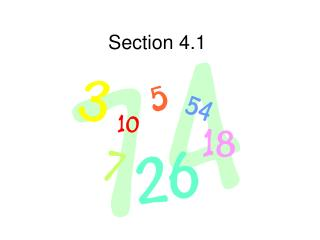DownloadDownload PresentationSection 4.1

# Section 4.1

Télécharger la présentation## Section 4.1

- - - - - - - - - - - - - - - - - - - - - - - - - - - E N D - - - - - - - - - - - - - - - - - - - - - - - - - - -
##### Presentation Transcript

1. Section 4.1

2. Radian and Degree Measure • The angles in Quadrant I are between 0 and 90 degrees. • The angles in Quadrant II are between 90 and 180 degrees. • The angles in Quadrant III are between 180 and 270 degrees. • The angles in Quadrant IV are between 270 and 360 degrees.

3. Radian and Degree Measure • Positive angles go counterclockwise. • Negative angles go clockwise. • Quadrant I is between 0 and pi/2 • Quadrant II is pi/2 and pi • Quadrant III is between pi and (3pi)/2 • Quadrant IV is between (3pi)/2 and 2pi

4. Radian and Degree Measure • To convert from degrees to radians you multiply by (pi)/180 • To convert from radians to degrees you multiply by 180/pi • Coterminal Angles: add or subtract 360 degrees Add or subtract 2pi

5. Radian and Degree Measure • Complement: 90 degrees minus the angle Pi/2 minus the angle • Supplement: 180 degrees minus the angle Pi minus the angle

6. Radian and Degree Measure • Arc Length: s = r (theta) • Theta must be in radians.